## Measurement: Volume of Cubes and Cuboids

Practice Unlimited Questions

#### Word Problem 1. What is the unknown edge of each of the cuboids below?

a)   Volume = 600 cm3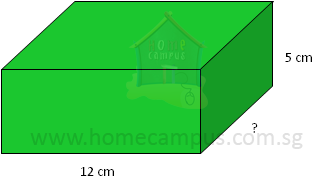Volume  =  Length × Breadth × Height

600  =  12 × Breadth × 5

 600 12 x 5

10 cm
b)   Volume = 4500 cm3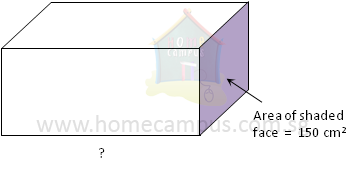Volume  =  Length × (Breadth × Height)

= Length × (Area of shaded face)

4500  =  Length × 150

Length  =
 4500 150

30 cm
c)   Volume = 6750 cm3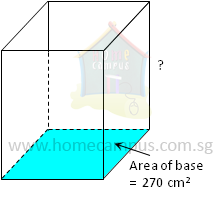Volume  =  Area of base × Height

6750 cm3 =  270 cm2 × Height

Height  =
 6750 270

25 cm

#### Word Problem 2: The volume of the Rubik's cube below is 216 cm3. What is the length of one edge of the cube?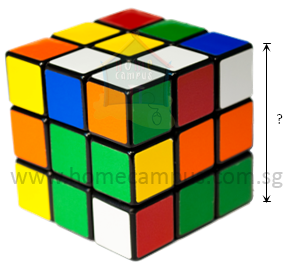The symbol ∛ stands for cube root.
∛(216) is read as the cube root of 216.

6 × 6 × 6  =  216
So, ∛(216) = 6
Length   =   ∛(216)

=  6 cm

The length of one edge of the Rubik's cube is 6 cm.

#### Word Problem 3: A rectangular container with a base area of 200 cm2 is filled with water to a height of 15 cm. If the container has a height of 20 cm, how much water (in litres) should be added to it to fill it completely?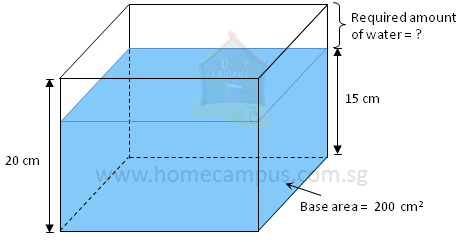Capacity of container   =   Base area × Height of container

=  200 cm2 × 20 cm

=  4000 cm3

=  4 litres
Volume of water   =   Base area × Height of water level

=  200 cm2 × 15 cm

=  3000 cm3

=  3 litres
4 litres − 3 litres   =  1 litre
1 litre water should be added to the container to fill it completely.

#### Word Problem 4: Josh built a rectangular cardboard box 18 cm high with a square base and a volume of 2178 cm3. Then he realized he did not need a box that large, so, he chopped off the height of the box reducing its volume to 1331 cm3. Was the new box cubical?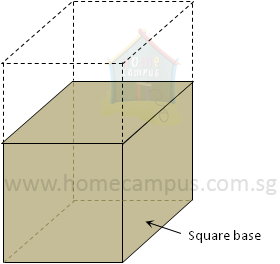Square base means Length = Breadth
Volume   =   (Length × Breadth) × Height

=  Base area × Height
Base area   =
 2178 18

=  121 cm2

The symbol √ stands for square root.

11 × 11 = 121
So, √121 = 11
Length × Length  =  121 cm2

Length = √(121)

11 cm
Now,

1331 cm3  =  11 cm × 11 cm × new height

New height  =
 1331 11 x 11

11 cm
Yes, the new box was cubical (as the length, width and height are all same).

#### Word Problem 5: A rectangular juice dispenser has a base area of 450 cm2 and a height of 25 cm. Gale adds syrup and water in the ratio 1 : 3 to the dispenser to make a beverage. If he uses 6.75 litres of water, what percentage of the dispenser will be filled when the beverage is made?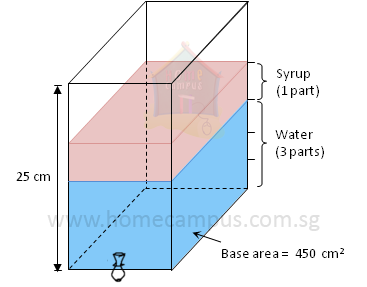Capacity of dispenser   =   Base area × Height of dispenser

= 450 cm2 × 25 cm

11250 cm3

Syrup : Water = 1 : 3
Volume of water  =  6.75 litres

Volume of syrup  =
 1 3
× 6.75 litres

=  2.25 litres
Total volume of beverage  =  6.75 litres + 2.25 litres

=  9 litres

9000 cm3
 9000 11250
× 100  =  80 %

80% of the dispenser will be filled when the beverage is made.

#### Word Problem 6: A rectangular aquarium is 2 5 filled. When 16 litres of water is added, the aquarium is 2 3 filled. Find the height of the aquarium if its length and width are 50 cm and 40 cm respectively.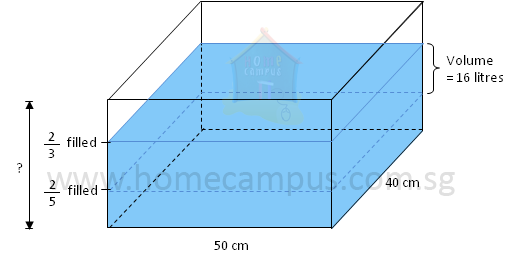2 3
 2 5
&nsbp;=
 4 15
So,

 4 15
of the capacity of the aquarium = 16 litres

 4 15
× the capacity of the aquarium = 16 litres
Then,

Capacity of the aquarium  =  16 litres ÷
 4 15

=  16 litres ×
 15 4

=  60 litres

6000 cm3
Therefore,

Height of the aquarium  =
 60000 50 x 40

30 cm

The height of the aquarium is 30 cm.

#### Word Problem 7: The water level in a rectangular tank 65 cm long and 45 cm wide is 14 cm. It will take another 58.5 litres of water to fill the tank to its brim. Find the height of the tank.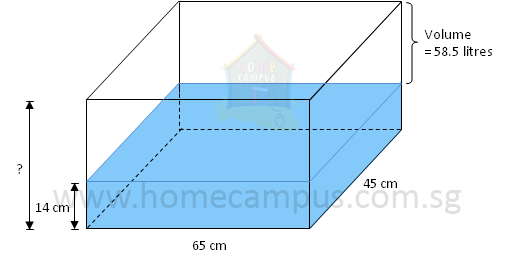Volume of water in the tank  =  65 cm × 45 cm × 14 cm

= 40950 cm3

40.95 litres
Capacity of the tank  =  40.95 litres + 58.5 litres

= 99.45 litres

99450 cm3
Height of the tank  =
 99450 65 x 45

34 cm

The height of the tank is 34 cm.

#### Word Problem 8: Containers A (6 cm, 5cm, 4cm), B (5 cm, 4 cm, 3 cm) and C (3 cm, 3 cm, 2 cm) are three rectangular containers. At first, Container A is filled with water to its brim while containers B and C are empty. Next, some water from Container A is poured into containers B and C so that Container B is completely full while Container C is half full. Find the height of the water left in Container A.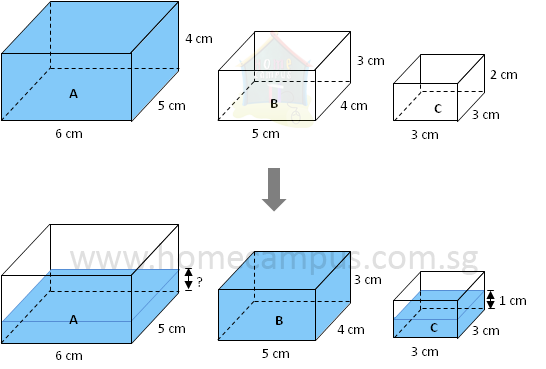Before

Volume of water in container A  =  6 cm × 5 cm × 4 cm

120 cm3
After

Volume of water in container B  =  5 cm × 4 cm × 3 cm

60 cm3

Volume of water in container C  =  3 cm × 3 cm × 1 cm

9 cm3
Volume of water left in container A  =  120 cm3 − 60 cm3 − 9 cm3

51 cm3
Height of water left in container A  =
 51 6 x 5

1.7 cm

The height of the water left in Container A is 1.7 cm.

#### Word Problem 9: A rectangular vase with a square base and 25 cm height is filled with water to its maximum capacity of 1.6 litres. Shelly puts some marbles into the vase causing 235 ml of water to spill. Then she removes all the marbles from the vase causing a further spillage of 85 ml of water. What is the height of the water level in the vase now?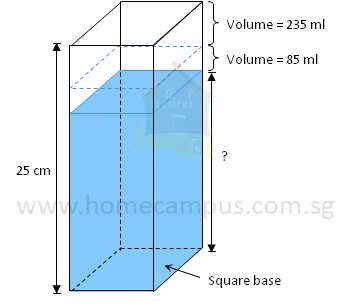Before the marbles are added to the vase

Volume of water in vase  =  1.6 litres

= 1600 cm3
Base area of vase  =
 1600 25

= 64 cm2
1.6 litres = 1600 ml
After the marbles are added to the vase

Volume of water in vase  =  1600 ml − 235 ml

= 1365 ml
After the marbles are removed from the vase

Volume of water in vase  =  1365 ml − 85 ml

= 1280 ml

= 1280 cm3
Height of the water level  =
 Volume Base Area

 1280 64

= 20 cm

The height of the water level in the vase is 20 cm now.

#### Word Problem 10: An empty rectangular bath tub 150 cm long, 60 cm wide and 50 cm high is being filled with water from a tap at a rate of 30 litres per minute. The tap is turned off after 12 minutes. Water is then drained out of the tub at a rate of 18 litres per minute. What would be the drop in the water level (measured in cm) 6 mintues later?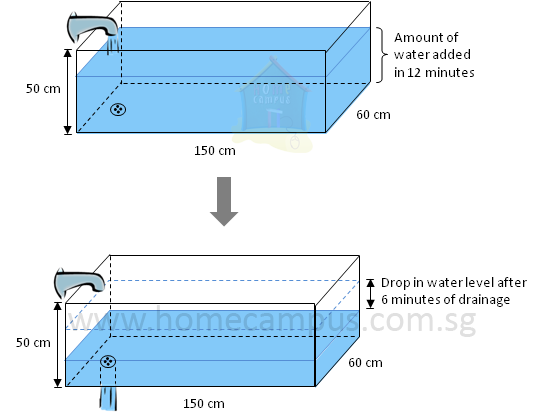Amount of water added to tub in 1 minute  =  30 litres

= Amount of water added to tub in 12 minutes  =  30 litres × 12

= 360 litres

360000 cm3
Height of the water level in tub at first  =
 360000 150 x 60

40 cm
Amount of water drained out of tub in 1 minute  =  18 litres

Amount of water drained out of tub in 6 minutes  =  18 litres × 6

= 108 litres

108000 cm3
Amount of water left in tub  =  360000 cm3 − 108000 cm3

252000 cm3
Height of the water level in tub 6 minutes later  =
 252000 150 x 60

28 cm
Difference in heights  =  40 cm − 28 cm

12 cm

The drop in the water level 6 minutes later would be 12 cm.

#### Word Problem 11: At 9 am, Kate turned on the supply tap which dispensed water at a rate of 3 litres per minute to fill an empty rectangular container. At the same time, water began leaking out of the container at a rate of 0.5 litres per minute through its faulty tap. Kate turned off the supply tap when the container was filled to its capacity. Given that the container measured 20 cm by 15 cm by 25 cm, at what time was the container completely empty again?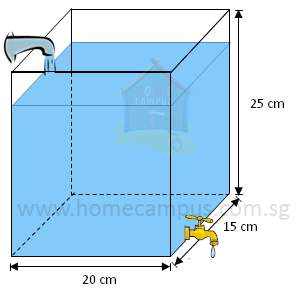Volume of container  =  20 cm × 15 cm × 25 cm

= 7500 cm3

7.5 litres
While the supply tap is turned on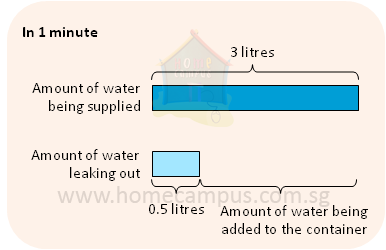Amount of water being added to the container in 1 minute

=  amount of water being supplied − amount of water leaking out

=  3 litres − 0.5 litres

=  2.5 litres
Time taken to fill the container completely

=
 7.5 2.5

=  3 minutes

3 minutes after 9 am is 9:03 am.

At 9:03 am, the container was filled to its capacity.
When the supply tap is turned off

Amount of water leaking out of the container in 1 minute

=  0.5 litres
Time taken to empty the container completely

=
 7.5 0.5

=  15 minutes

15 minutes after 9:03 am is 9:18 am.

At 9:18 am, the container was completely empty again.